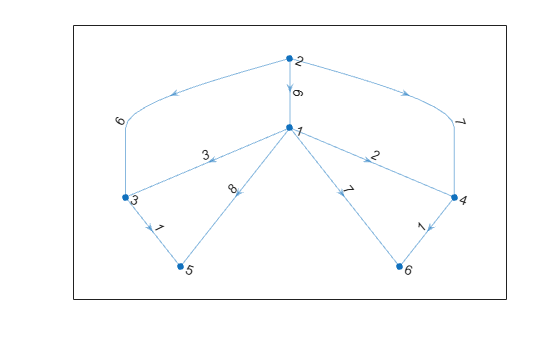reordernodes

Reorder graph nodes

Description

example

H = reordernodes(G,order) reorders the nodes in graph G according to order. This reordering corresponds to a symmetric permutation of the adjacency matrix of G.

example

[H,idx] = reordernodes(G,order) also returns the permutation vector for the edge indices, idx. For example, if G.Edges has a variable Weight, then H.Edges.Weight == G.Edges.Weight(idx).

Examples

collapse all

Create and plot a graph.

s = [1 1 1 2 5 3 6 4 7 8 8 8];
t = [2 3 4 5 3 6 4 7 2 6 7 5];
G = graph(s,t);
plot(G)Reorder the graph nodes using a permutation vector.

order = [7 2 3 4 8 1 5 6];
G = reordernodes(G,order);
plot(G)Create and plot a weighted graph.

s = [1 1 1 2 2 2 2 3 4];
t = [3 4 2 3 4 5 6 5 6];
weights = [6 7 6 3 2 8 7 1 1];
G = digraph(s,t,weights);
plot(G,'EdgeLabel',G.Edges.Weight)Reorder the graph nodes based on the out-degree, such that node 1 has the largest out-degree.

[~,order] = sort(outdegree(G),'descend')
order = 6×1

2
1
3
4
5
6

[H,idx] = reordernodes(G,order);
plot(H,'EdgeLabel',H.Edges.Weight)idx describes the permutation of the rows in G.Edges. Confirm this correspondence using the Weight variable.

isequal(H.Edges.Weight, G.Edges.Weight(idx))
ans = logical
1

Input Arguments

collapse all

Input graph, specified as either a graph or digraph object. Use graph to create an undirected graph or digraph to create a directed graph.

Example: G = graph(1,2)

Example: G = digraph([1 2],[2 3])

Node order, specified as node indices or node names. order specifies a symmetric permutation of the adjacency matrix of G. If A = adjacency(G), then A(order,order) produces adjacency(H).

order can be one of:

• Numeric vector of node indices, such as [1 3 2].

• A cell array of character vectors or string array of node names, such as {'A' 'C' 'B'} or ["A" "C" "B"].

Example: H = reordernodes(G,[3 1 2])

Output Arguments

collapse all

Output graph, returned as a graph or digraph object. H contains the same Nodes and Edges properties as G, but with the rows of H.Nodes and H.Edges permuted:

• H.Nodes is the same as G.Nodes(order,:).

• H.Edges is similar to G.Edges(idx,:), except that the nodes are renumbered.

Permutation vector of edge indices, returned as a vector. The values in idx describe the permutation of the rows in G.Edges.